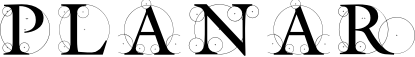#### Previous topic

planar.BoundingBox – Bounding Boxes

#### Next topic

Release 0.3 (2/26/2011)

# planar.Polygon – Polygonal Shapes¶

class planar.Polygon(vertices, is_convex=None, is_simple=None)

Arbitrary polygon represented as a list of vertices.

The individual vertices of a polygon are mutable, but the number of vertices is fixed at construction.

Parameters: vertices – Iterable containing three or more Vec2 objects. is_convex (bool) – Optionally allows the polygon to be declared convex or non-convex at construction time, thus saving additional time spent checking the vertices to calculate this property later. Only specify this value if you are certain of the convexity of the vertices provided, as no additional checking will be performed. The results are undefined if a non-convex polygon is declared convex or vice-versa. Note that triangles are always considered convex, regardless of this value. is_simple (bool) – Optionally allows the polygon to be declared simple (i.e., not self-intersecting) or non-simple at construction time, which can save time calculating this property later. As with is_convex above, only specify this value if you are certain of this value for the vertices provided, or the results are undefined. Note that convex polygons are always considered simple, regardless of this value.

Note

Several operations on polygons, such as checking for containment, or intersection, rely on knowing the convexity to select the appropriate algorithm. So, it may be beneficial to specify these values in the constructor, even if your application does not access the is_convex, or is_simple properties itself later. However, be cautious when specifying these values here, as incorrect values will likely result in incorrect results when operating on the polygon.

Note

If the polygon is mutated, the cached values of is_convex and is_simple will be invalidated.

almost_equals(other)

Compare for approximate equality.

contains_point(point)

Return True if the specified point is inside the polygon.

This test can use various strategies depending on the classification of the polygon, i.e., triangular, radial, y-monotone, convex, or other.

The runtime complexity will depend on the polygon:

Triangle or best-case radial: O(1) y-monotone, convex: O(log n) other: O(n)

Parameters: point (Vec2) – A point vector. bool
classmethod convex_hull(points)

Return a new polygon that is the convex hull of the supplied sequence of points.

If points is a polygon known to be convex, a copy of the polygon is returned.

The hull is computed using an adaptive quick-hull algorithm. The expected runtime complexity of this algorithm is O(n log h) (where h is the size of the hull), the worst case is O(n log n) when the supplied points are already nearly convex. This algorithm is especially fast when many of the supplied points are inside the resulting hull.

Parameters: points – A sequence of points. Polygon
classmethod from_points(points)

Create a polygon from a sequence of points

classmethod regular(vertex_count, radius, center=(0, 0), angle=0)

Create a regular polygon with the specified number of vertices radius distance from the center point. Regular polygons are always convex.

Parameters: vertex_count (int) – The number of vertices in the polygon. Must be >= 3. radius (float) – distance from vertices to center point. center (Vec2) – The center point of the polygon. If omitted, the polygon will be centered on the origin. angle (float) – The starting angle for the vertices, in degrees.

Create a radial pointed star polygon with the specified number of peaks.

Parameters: peak_count (int) – The number of peaks. The resulting polygon will have twice this number of vertices. Must be >= 2. radius1 (float) – The peak or valley vertex radius. A vertex is aligned on angle with this radius. radius2 (float) – The alternating vertex radius. center (Vec2) – The center point of the polygon. If omitted, the polygon will be centered on the origin. angle (float) – The starting angle for the vertices, in degrees.
tangents_to_point(point)

Given a point exterior to the polygon, return the pair of vertex points from the polygon that define the tangent lines with the specified point.

Runtime Complexity: O(log n) convex, O(n) other

Parameters: point (Vec2) – A point outside the polygon. If the point specified is inside, the result is undefined. A tuple containing the left and right tangent points. tuple of Vec2
bounding_box

The bounding box of the polygon

centroid

The geometric center point of the polygon. This point only exists for simple polygons. For non-simple polygons it is None. Note in concave polygons, this point may lie outside of the polygon itself.

If the centroid is unknown, it is calculated from the vertices and cached. If the polygon is known to be simple, this takes O(n) time. If not, then the simple polygon check is also performed, which has an expected complexity of O(n log n).

is_centroid_known

True if the polygon’s centroid has been pre-calculated and cached.

Mutating the polygon will invalidate the cached value.

is_convex

True if the polygon is convex.

If this is unknown then it is calculated from the vertices of the polygon and cached. Runtime complexity: O(n)

is_convex_known

True if the polygon is already known to be convex or not.

If this value is True, then the value of is_convex is cached and does not require additional calculation to access. Mutating the polygon will invalidate the cached value.

is_simple

True if the polygon is simple, i.e., it has no self-intersections.

If this is unknown then it is calculated from the vertices of the polygon and cached. Runtime complexity: O(n) convex, O(n log n) expected for most non-convex cases, O(n^2) worst case non-convex

is_simple_known

True if the polygon is already known to be simple or not.

If this value is True, then the value of is_simple is cached and does not require additional calculation to access. Mutating the polygon will invalidate the cached value.